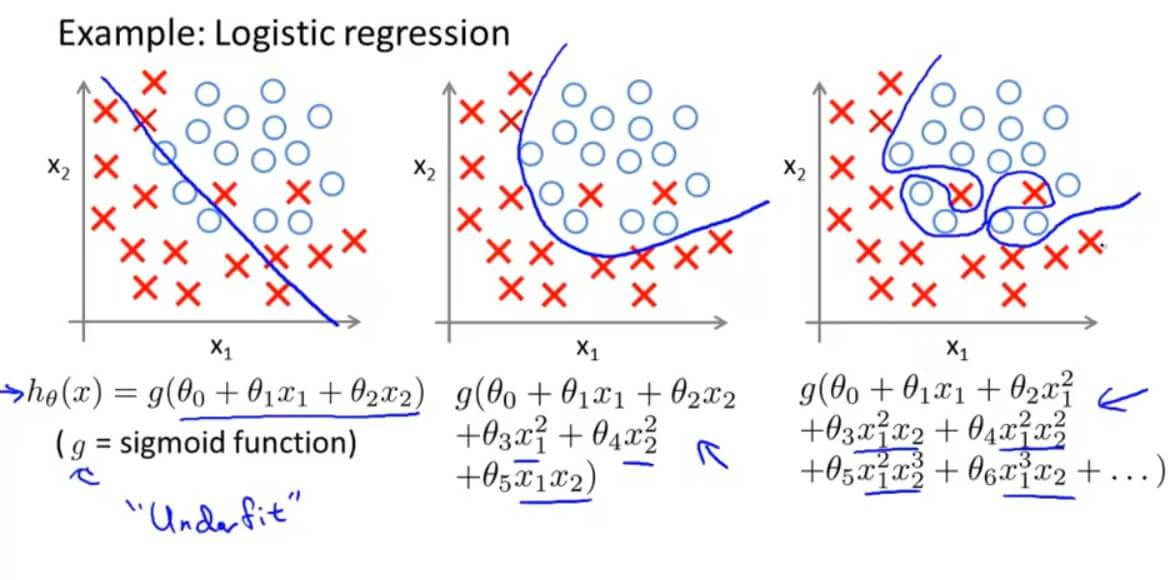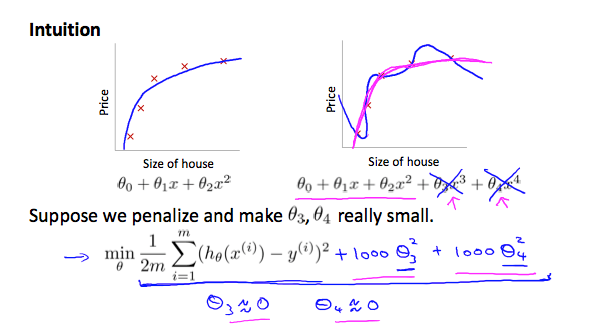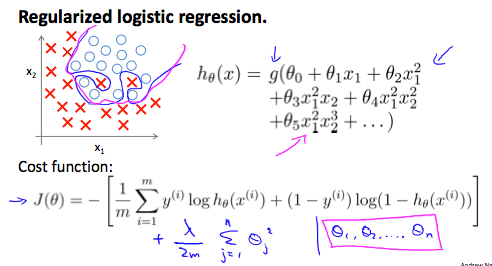### Discover more content...

Enter some keywords in the search box above, we will do our best to offer you relevant results.

### We're sorry!

We couldn't find any results for your search. Please try again with another keywords.

## 一. Solving the Problem of Overfitting### 1. 减少特征的数量：

• 手动选择要保留的特征，哪些变量更为重要，哪些变量应该保留，哪些应该舍弃。
• 使用模型选择算法（稍后在课程中学习），算法会自动选择哪些特征变量保留，哪些舍弃。

### 2. 正则化

• 保留所有的特征，但减少参数 $\theta_{j}$ 的大小或者减少量级。
• 当有很多个特征的时候，并且每个特征都会对最终预测值产生影响，正则化可以保证运作良好。$$\rm{CostFunction} = \rm{F}({\theta}) = \frac{1}{2m} \left [ \sum_{i = 1}^{m} (h_{\theta}(x^{(i)})-y^{(i)})^2 + \lambda \sum_{i = 1}^{m} \theta_{j}^{2} \right ]$$

$\lambda \sum_{i = 1}^{m} \theta_{j}^{2}$ 是正则化项，它缩小每个参数的值。 $\lambda$ 是正则化参数，$\lambda$ 控制两个不同目标之间的取舍，即更好的去拟合训练集的目标 和 将参数控制的更小的目标，从而保持假设模型的相对简单，避免出现过拟合的情况。

## 二. Regularized Linear Regression 线性回归正则化

### 1. Gradient Descent 线性回归梯度下降正则化

$$\theta_{0} := \theta_{0} - \alpha \frac{1}{m} \sum_{i = 1}^{m} (h_{\theta}(x^{(i)})-y^{(i)})x_{0}^{(i)}$$
$$\theta_{j} := \theta_{j} - \alpha \left [ \left ( \frac{1}{m} \sum_{i = 1}^{m} (h_{\theta}(x^{(i)})-y^{(i)})x_{j}^{(i)}\right ) + \frac{\lambda}{m}\theta_{j} \right ] \;\;\;\;\;\;\;\;j \in \begin{Bmatrix} 1,2,3,4, \cdots n\end{Bmatrix}$$

$$\theta_{j} := \theta_{j}(1-\alpha \frac{\lambda}{m}) - \alpha \frac{1}{m} \sum_{i = 1}^{m} (h_{\theta}(x^{(i)})-y^{(i)})x_{j}^{(i)} \;\;\;\;\;\;\;\;j \in \begin{Bmatrix} 1,2,3,4, \cdots n\end{Bmatrix}$$

### 2. Normal Equation 线性回归正规方程正则化

$$\Theta = (X^{T}X)^{-1}X^{T}Y$$

$$\Theta = \left( X^{T}X +\lambda \begin{bmatrix} 0 & & & & \\ & 1 & & & \\ & & 1 & & \\ & & & \ddots & \\ & & & & 1 \end{bmatrix} \right) ^{-1}X^{T}Y$$

## 三. Regularized Logistic Regression 逻辑回归正则化\begin{align*} \rm{CostFunction} = \rm{F}({\theta}) &= -\frac{1}{m}\left [ \sum_{i=1}^{m} y^{(i)}logh_{\theta}(x^{(i)}) + (1-y^{(i)})log(1-h_{\theta}(x^{(i)})) \right ] \\ \left( h_{\theta}(x) = \frac{1}{1+e^{-\theta^{T}x}} \right ) \end{align*}

\begin{align*} \rm{CostFunction} = \rm{F}({\theta}) &= -\frac{1}{m}\left [ \sum_{i=1}^{m} y^{(i)}logh_{\theta}(x^{(i)}) + (1-y^{(i)})log(1-h_{\theta}(x^{(i)})) \right ] +\frac{\lambda}{2m} \sum_{j=1}^{n}\theta_{j}^{2} \\ \end{align*}

### 1. Gradient Descent 逻辑回归梯度下降正则化

\begin{align*} \theta_{0} &:= \theta_{0} - \alpha \frac{1}{m} \sum_{i = 1}^{m} (h_{\theta}(x^{(i)})-y^{(i)})x_{0}^{(i)} \;\;\;\;\;\;\;\;\;\;\;\;\;\;\;\;\;\;\;\;\;j = 1 \\ \theta_{j} &:= \theta_{j}(1-\alpha \frac{\lambda}{m}) - \alpha \frac{1}{m} \sum_{i = 1}^{m} (h_{\theta}(x^{(i)})-y^{(i)})x_{j}^{(i)} \;\;\;\;\;\;\;\;j \in \begin{Bmatrix} 1,2,3,4, \cdots n\end{Bmatrix} \\ \end{align*}

$$h_{\theta}(x) = \frac{1}{1+e^{-\theta^{T}x}}$$

## 四. Regularization 测试

### 1. Question 1

You are training a classification model with logistic regression. Which of the following statements are true? Check all that apply.

A. Introducing regularization to the model always results in equal or better performance on the training set.

B. Introducing regularization to the model always results in equal or better performance on examples not in the training set.

C. Adding many new features to the model makes it more likely to overfit the training set.

D. Adding a new feature to the model always results in equal or better performance on examples not in the training set.

A、B 正则化的引入是解决过拟合的问题，而过拟合正是过度拟合数据但无法泛化到新的数据样本中。
D 增加一些特征量可能导致拟合在训练集原本没有被拟合到的数据，正确，这就是过拟合。

### 2. Question 2

Suppose you ran logistic regression twice, once with λ=0, and once with λ=1. One of the times, you got

parameters $\theta = \begin{bmatrix} 26.29\\ 65.41 \end{bmatrix}$, and the other time you got $\theta = \begin{bmatrix} 2.75\\ 1.32 \end{bmatrix}$. However, you forgot which value of λ corresponds to which value of θ. Which one do you think corresponds to λ=1?

A. $\theta = \begin{bmatrix} 26.29\\ 65.41 \end{bmatrix}$

B. $\theta = \begin{bmatrix} 2.75\\ 1.32 \end{bmatrix}$

$\lambda = 1$表示正则化以后。正则化其实让我们的 $\theta_j$变小，所以选B。

### 3. Question 3

Which of the following statements about regularization are true? Check all that apply.

A. Using too large a value of λ can cause your hypothesis to overfit the data; this can be avoided by reducing λ.

B. Consider a classification problem. Adding regularization may cause your classifier to incorrectly classify some training examples (which it had correctly classified when not using regularization, i.e. when λ=0).

C. Because logistic regression outputs values 0≤hθ(x)≤1, its range of output values can only be "shrunk" slightly by regularization anyway, so regularization is generally not helpful for it.

D. Using a very large value of λ cannot hurt the performance of your hypothesis; the only reason we do not set λ to be too large is to avoid numerical problems.

C 正则化对逻辑回归没用，错误。
A、D $\lambda$过大会导致欠拟合。

GitHub Repo：Halfrost-Field

Follow: halfrost · GitHubPrevious Post

Next Post